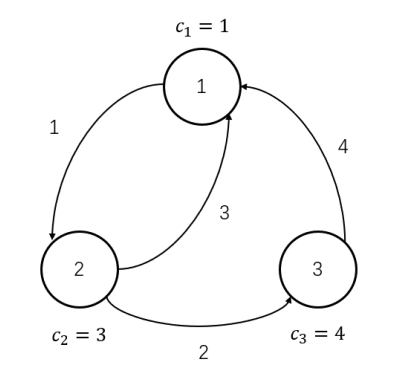# #558. 【NOI2020】美食家• 第 0 天，小 W 从城市 1 开始旅行，获得愉悦值 1 并向城市 2 出发。
• 第 1 天，小 W 到达城市 2，获得愉悦值 3 并向城市 1 出发。
• 第 4 天，小 W 到达城市 1，获得愉悦值 1 并向城市 2 出发。
• 第 5 天，小 W 到达城市 2，获得愉悦值 3 并向城市 3 出发。
• 第 7 天，小 W 到达城市 3，获得愉悦值 4 并向城市 1 出发。
• 第 11 天，小 W 到达城市 1，获得愉悦值 1 并结束旅行。
• 小 W 在该旅行中获得的愉悦值之和为 13。

### 输入格式

• 对所有 $i$，有 $u_i \neq v_i$。但数据中可能存在路线重复的单向道路，即可能存在 $1 \le i < j \le m$，使得 $u_i = u_j,v_i = v_j$。
• 对每座城市都满足：至少存在一条以该城市为起点的单向道路。
• 每次美食节的举办时间 $t_i$ 互不相同。

### 样例一

#### input

3 4 11 0
1 3 4
1 2 1
2 1 3
2 3 2
3 1 4


#### output

13


### 样例二

#### input

4 8 16 3
3 1 2 4
1 2 1
1 3 1
1 3 2
3 4 3
2 3 2
3 2 1
4 2 1
4 1 5
3 3 5
1 2 5
5 4 20


#### output

39


#### explanation

• 第 $0$ 天，小 W 从城市 $1$ 开始旅行，获得愉悦值 $3$ 并沿道路 $3$ 通行。
• 第 $2$ 天，小 W 到达城市 $3$，获得愉悦值 $2$ 并沿道路 $4$ 通行。
• 第 $5$ 天，小 W 到达城市 $4$，由于美食节获得愉悦值 $20+4$ 并沿道路 $7$ 通行。
• 第 $6$ 天，小 W 到达城市 $2$，获得愉悦值 $1$ 并沿道路 $5$ 通行。
• 第 $8$ 天，小 W 到达城市 $3$，获得愉悦值 $2$ 并沿道路 $4$ 通行。
• 第 $11$ 天，小 W 到达城市 $4$，获得愉悦值 $4$ 并沿道路 $8$ 通行。
• 第 $16$ 天，小 W 到达城市 $1$，获得愉悦值 $3$ 并结束旅行。
• 小 W 获得的愉悦值之和为 $39$。

### 数据范围

$1 \le n \le 50,n \le m \le 501,0 \le k \le 200,1 \le t_i \le T \le 10^9$.

$1 \le w_i \le 5,1 \le c_i \le 52501,1 \le u_i,v_i,x_i \le n,1 \le y_i \le 10^9$.

$1 \sim 4$$\le 5$$\le 50$$\le 5 5 \sim 8$$\le 50$$\le 52501 9 \sim 10$$\le 10^9$A
$11 \sim 13$$k=0 14 \sim 15$$k \le 10$
$16 \sim 17$
$18 \sim 20$$\le 501$